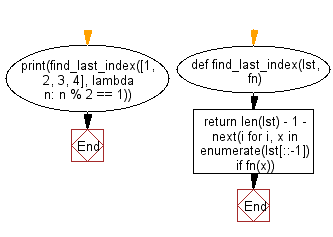﻿ Python: Find the index of the last element in the given list that satisfies a testing function - w3resource

# Python: Find the index of the last element in the given list that satisfies a testing function

## Python List: Exercise - 235 with Solution

Write a Python program to find the index of the last element in the given list that satisfies the provided testing function.

Sample Solution:

Python Code:

``````def find_last_index(lst, fn):
return len(lst) - 1 - next(i for i, x in enumerate(lst[::-1]) if fn(x))

print(find_last_index([1, 2, 3, 4], lambda n: n % 2 == 1))
```
```

Sample Output:

```2
```

Flowchart:## Visualize Python code execution:

The following tool visualize what the computer is doing step-by-step as it executes the said program:

Python Code Editor:

Have another way to solve this solution? Contribute your code (and comments) through Disqus.

What is the difficulty level of this exercise?

Test your Programming skills with w3resource's quiz.

﻿

## Python: Tips of the Day

Returns True if the provided function returns True for every element in the list, False otherwise:

Example:

```def tips_every(lst, fn=lambda x: x):
return all(map(fn, lst))

print(tips_every([2, 4, 3], lambda x: x > 1))
print(tips_every([1, 2, 3]))
```

Output:

```True
True
```